Select Page

# CBSE MCQ Maths 12 Science Vector Algebra Solutions in English

CBSE MCQ Maths 12 Science Vector Algebra Solutions in English to enable students to get Solutions in a narrative video format for the specific question.

Expert Teacher provides CBSE MCQ Maths 12 Science Vector Algebra Solutions through Video Solutions in English language. This video solution will be useful for students to understand how to write an answer in exam in order to score more marks. This teacher uses a narrative style for a question from Vector Algebra not only to explain the proper method of answering question, but deriving right answer too.

Please find the question below and view the Solution in a narrative video format.

Question:

Solution Video in English:

You can select video Solutions from other languages also. Please check Solutions in ( Hindi )

## Similar Questions from CBSE, 12th Science, Maths, Vector Algebra

Question 1 : Find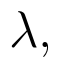if the vectors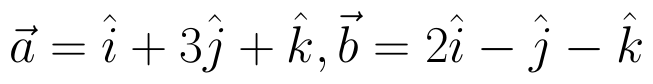and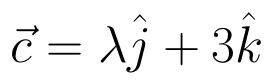are coplanar.   (View Answer Video)

Question 2 : Find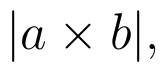if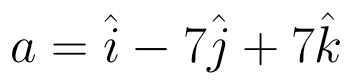and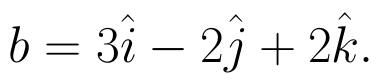(View Answer Video)

Question 3 :  Find a vector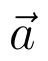of magnitude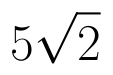making an angle of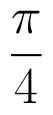with x-axis,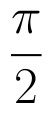with y-axis and an acute angle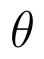with z-axis.  (View Answer Video)

Question 4 : If the vertices A, B, C of a triangle ABC have position vectors (1, 2, 3), (-1, 0, 0), (0, 1, 2) respectively then find <ABC (<ABC is the angle between the vectors BA and BC). (View Answer Video)

Question 5 : Find a unit vector parallel to the sum of vectors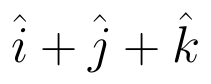and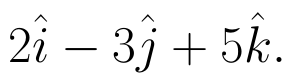(View Answer Video)

### Differential Equations

Question 1 :  Find the particular solution of the differential equation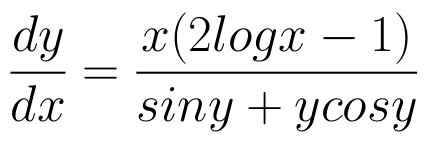given that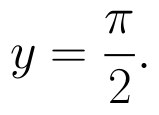When x =1.  (View Answer Video)

Question 2 : Obtain the differential equation of the family of circles passing through the points (a, 0) and (-a, 0). (View Answer Video)

Question 3 : Write the differential equation representing the curve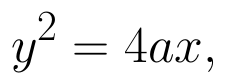where a is an arbitrary constant. (View Answer Video)

Question 4 : Find the general solution of the following differential equation :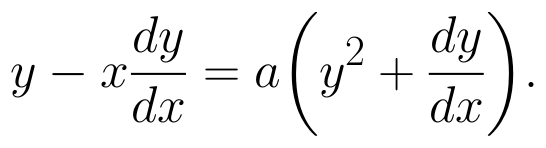(View Answer Video)

Question 5 : Write the differential equation formed from the equation y = mx + c, where m and c are arbitrary constants.

### Relations and Functions

Question 1 : Let * be the binary operation on N given by a * b = LCM of a and b. Find 20 * 16. (View Answer Video)

Question 2 : Let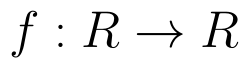be defined as f(x) = 3x.  (View Answer Video)

Question 3 : The law a + b = b + a is called ______. (View Answer Video)

Question 4 : If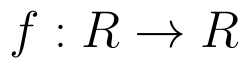is defined by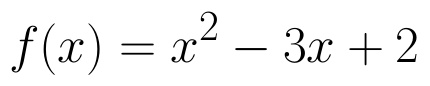, write f(f(x)). (View Answer Video)

Question 5 : Functions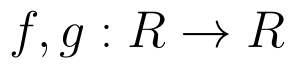are defined respectively, by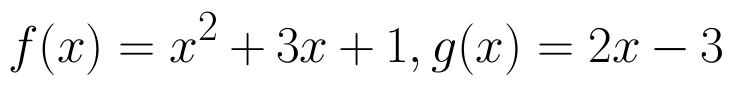, find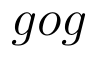(View Answer Video)

### Determinant

Question 1 : Find the value of k, if the area of the triangle is 4 sq unit and vertices are (k, 0) (4, 0) (0, 2). (View Answer Video)

Question 2 : If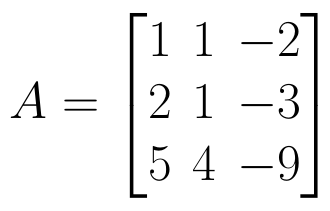find |A|. (View Answer Video)

Question 3 : Find the equation of the line joining (1, 2) and (3, 6) using determinants. (View Answer Video)

Question 4 : Let A be a non-singular square matrix of order 3 * 3. Then | adj A | is equal to: (View Answer Video)

Question 5 :  Find the area of the triangle with vertices at the points (-2, -3), (3, 2), (-1, -8). (View Answer Video)